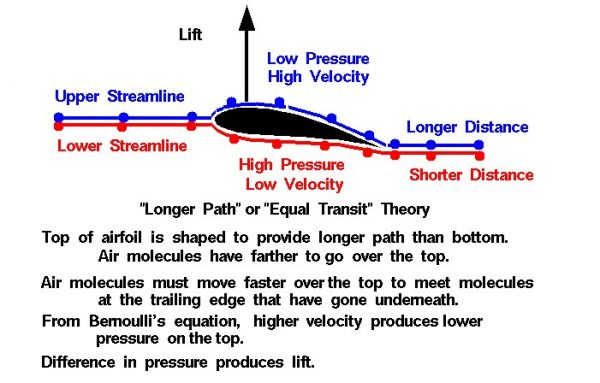# Equal Transit Theory InteractiveThere are many theories of how lift is generated. Unfortunately, many of the theories found in encyclopedias, on web sites, and even in some textbooks are incorrect, causing unnecessary confusion for students.

## Incorrect Airfoil Theory

The theory described on this slide is one of the most widely circulated, incorrect explanations. The theory can be labeled the “Longer Path” theory, or the “Equal Transit Time” theory. The theory states that airfoils are shaped with the upper surface longer than the bottom.

The air molecules (the little colored balls on the figure) have farther to travel over the top of the airfoil than along the bottom. In order to meet up at the trailing edge, the molecules going over the top of the wing must travel faster than the molecules moving under the wing. Because the upper flow is faster, then, from Bernoulli’s equation, the pressure is lower. The difference in pressure across the airfoil produces the lift.

### m dot = r * V * A = constant

For a constant density, decreasing the area increases the velocity.

Turning to the incorrect airfoil theory, the top of the airfoil is curved, which constricts the flow. Since the area is decreased, the velocity over the top of the foil is increased. Then from Bernoulli’s equation, higher velocity produces a lower pressure on the upper surface. The low pressure over the upper surface of the airfoil produces the lift.

Before considering what is wrong with this theory, let’s investigate the actual flow around an airfoil by doing a couple of experiments using a Java simulator which is solving the correct flow equations. Below the simulator is a text box with instructions. Be sure that the slider on the right of the text box is pulled to the top to begin the experiments

Let’s use the information we’ve just learned to evaluate the various parts of the “Equal Transit” Theory.

## Lifting airfoils are designed to have the upper surface longer than the bottom.

• This is not always correct. The symmetric airfoil in our experiment generates plenty of lift and its upper surface is the same length as the lower surface. Think of a paper airplane. Its airfoil is a flat plate –> top and bottom exactly the same length and shape and yet they fly just fine. This part of the theory probably got started because early airfoils were curved and shaped with a longer distance along the top. Such airfoils do produce a lot of lift and flow turning, but it is the turning that’s important, not the distance. There are modern, low-drag airfoils which produce lift on which the bottom surface is actually longer than the top. This theory also does not explain how airplanes can fly upside-down which happens often at air shows and in air-to-air combat. The longer surface is then on the bottom!

## Air molecules travel faster over the top to meet molecules moving underneath at the trailing edge.

• Experiment #1 shows us that the flow over the top of a lifting airfoil does travel faster than the flow beneath the airfoil. But the flow is much faster than the speed required to have the molecules meet up at the trailing edge. Two molecules near each other at the leading edge will not end up next to each other at the trailing edge as shown in Experiment #2. This part of the theory attempts to provide us with a value for the velocity over the top of the airfoil based on the non-physical assumption that the molecules meet at the aft end. We can calculate a velocity based on this assumption, and use Bernoulli’s equation to compute the pressure, and perform the pressure-area calculation and the answer we get does not agree with the lift that we measure for a given airfoil. The lift predicted by the “Equal Transit” theory is much less than the observed lift, because the velocity is too low. The actual velocity over the top of an airfoil is much faster than that predicted by the “Longer Path” theory and particles moving over the top arrive at the trailing edge before particles moving under the airfoil.

## The upper flow is faster and from Bernoulli’s equation the pressure is lower.

• The difference in pressure across the airfoil produces the lift. As we have seen in Experiment #1, this part of the theory is correct. In fact, this theory is very appealing because many parts of the theory are correct. In our discussions on pressure-area integration to determine the force on a body immersed in a fluid, we mentioned that if we know the velocity, we can obtain the pressure and determine the force. The problem with the “Equal Transit” theory is that it attempts to provide us with the velocity based on a non-physical assumption as discussed above.
Provide feedback